# Three Phase Permanent Magnet Synchronous Machine (VBR)

Description of the Three Phase Permanent Magnet Synchronous Machine (VBR) component in Schematic Editor.

Table 1. Three Phase Permanent Magnet Synchronous Machine (VBR) component in Typhoon HIL Schematic Editor
component component dialog window component parameters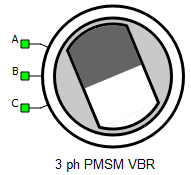• Property tabs:

A, B, and C are stator winding terminals. The stator winding uses the voltage behind reactance interface.

## Electrical sub-system model

The electrical part of the machine is represented by the following system of equations, modeled in the rotating dq reference frame. The dq reference frame is attached to the rotor, and the direct axis is aligned with the rotor magnets. The modeled dynamics can be represented with the following equations:

$\left[\begin{array}{c}{v}_{ds}\\ {v}_{qs}\end{array}\right]=\left[\begin{array}{cc}{R}_{s}& 0\\ 0& {R}_{s}\end{array}\right]\left[\begin{array}{c}{i}_{ds}\\ {i}_{qs}\end{array}\right]+\frac{d}{dt}\left[\begin{array}{c}{\psi }_{ds}\\ {\psi }_{qs}\end{array}\right]+{\omega }_{r}\left[\begin{array}{c}{-\psi }_{qs}\\ {\psi }_{ds}\end{array}\right]$

$\left[\begin{array}{c}{\psi }_{ds}\\ {\psi }_{qs}\end{array}\right]=\left[\begin{array}{cc}{L}_{d}& {0}_{}\\ {0}_{}& {L}_{q}\end{array}\right]\left[\begin{array}{c}{i}_{ds}\\ {i}_{qs}\end{array}\right]+\left[\begin{array}{c}{\psi }_{PM}\\ 0\end{array}\right]$

${T}_{e}=\frac{3}{2}p\left({\psi }_{ds}{i}_{qs}-{\psi }_{qs}{i}_{ds}\right)$

If the saturation effects are considered, the equations have the same form, but in that case fluxes are functions of stator currents:

Table 2. Three Phase Permanent Magnet Synchronous Machine electrical subsystem model variables
symbol description
ψds Direct axis component of the stator flux [Wb]
ψqs Quadrature axis component of the stator flux [Wb]
ψPM Flux amplitude established in stator phases by rotor permanent magnets [Wb]
ids Direct axis component of the stator current [A]
iqs Quadrature axis component of the stator current [A]
vds Direct axis component of the stator voltage [V]
vqs Quadrature axis component of the stator voltage [V]
Rs Stator phase resistance [Ω]
Ld Direct axis inductance [H]
ωr Rotor electrical speed [rad/s] ( $=p{\omega }_{m}$ )
p Machine number of pole pairs
Te Machine developed electromagnetic torque [Nm]

## Mechanical sub-system model

Motion equation:

Table 3. Mechanical sub-system model variables
symbol description
Jm Combined rotor and load moment of inertia [kgm2]
Te Machine developed electromagnetic torque [Nm]
Tl Shaft mechanical load torque [Nm]
b Machine viscous friction coefficient [Nms]
Note: Motion equation is the same for all of the rotating machine models.

## Electrical

This component offers two levels of model fidelity, designated by the Model Type property. The following options are available:
• linear
• nonlinear
If the selected Model Type is nonlinear, the following saturation types can be specified:
• flux vs current
• absolute inductance vs current
• incremental inductance vs current
Table 4. Electrical parameters
symbol description
Rs Stator phase resistance [Ω]
Ld Direct axis inductance [H]
Psi_pm Flux amplitude established in stator phases by rotor permanent magnets [Wb]
id vector List of stator current direct axis component values [A]
iq vector List of stator current quadrature axis component values [A]
psid table Table of stator flux direct axis component values [Wb]
psiq table Table of stator flux quadrature axis component values [Wb]
Ld table Table of stator inductance direct axis component values [H]
Lq table Table of stator inductance quadrature axis component values [H]

The permanent magnet synchronous machine VBR model can include magnetic saturation effects. In that case, fluxes or inductances are defined as functions of stator currents ids and iqs. These functions are represented in the form of lookup tables. The lookup tables use linear interpolation and linear extrapolation.

Saturation can be parametrized in the following ways:
1. fluxes vs stator currents
2. absolute inductances vs stator currents
3. incremental inductances vs stator currents
In each of these cases the direct axis and quadrature axis flux or inductance can depend on both stator currents or only on the corresponding stator current. When fluxes or inductances are functions of both direct axis and quadrature axis currents, it is important to define the lookup tables as nested Python lists where the number of inner lists must be equal to the number of elements in the current id vector and the number of elements of each inner list must also be equal to the number of elements in the current iq vector.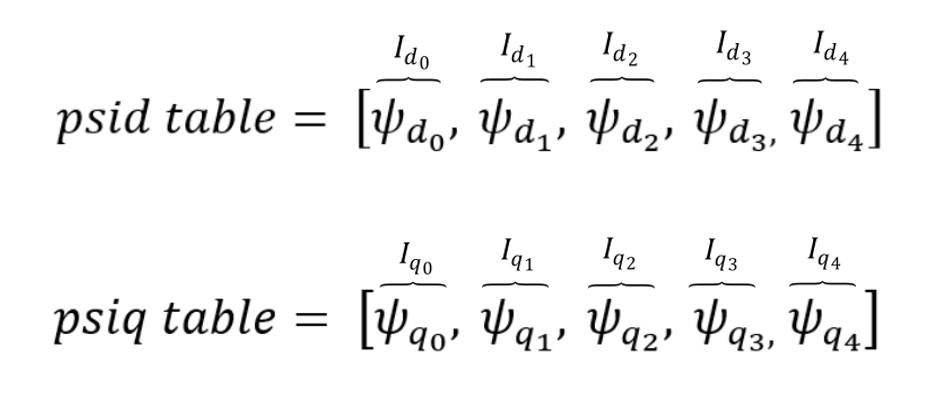Figure 1. Nonlinear Model Type: fluxes as functions of stator currents, ψd=fd(id) , ψq=fq(iq)
id_vector = [-40.0, -20.0, 0.0, 20.0, 40.0]
iq_vector = [-40.0, -20.0, 0.0, 20.0, 40.0]
psid_table = [-0.0492472, -0.0433668, -0.0425532, -0.0433464, -0.0484104]
psiq_table = [-0.1330824, -0.0838922, 0.0, 0.0838828, 0.133098]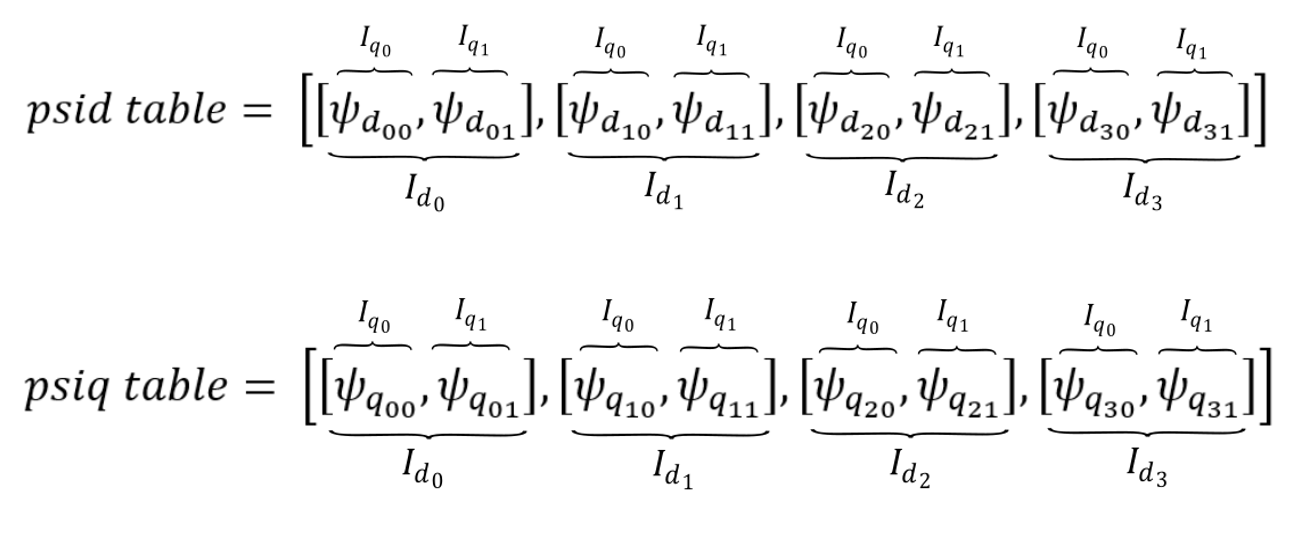Figure 2. Nonlinear Model Type: fluxes as functions of stator currents, ψd=fd(id , iq) , ψq=fq(id , iq)
id_vector = [-40.0, -20.0, 0.0, 20.0, 40.0]
iq_vector = [-40.0, -20.0, 0.0, 20.0, 40.0]
psid_table = [[-0.0492472, -0.0433668, -0.0425532, -0.0433464, -0.0484104],
[-0.0115952, -0.0274476, -0.0330376, -0.02771, -0.0126918],
[0.032, 0.032, 0.032, 0.032, 0.032],
[0.064706, 0.0662274, 0.0593586, 0.0677826, 0.0649068],
[0.0805368, 0.0705448, 0.05448328, 0.070713, 0.0812716]]
psiq_table = [[-0.1330824, -0.0838922, 0.0, 0.0838828, 0.133098],
[-0.1313616, -0.1041012, 0.0, 0.1041148, 0.1282268],
[-0.1286288, -0.1076058, 0.0, 0.107, 0.1278272],
[-0.1175936, -0.084391, 0.0, 0.0839394, 0.1162836],
[-0.1092448, -0.0588548, 0.0, 0.0585804, 0.1084576]]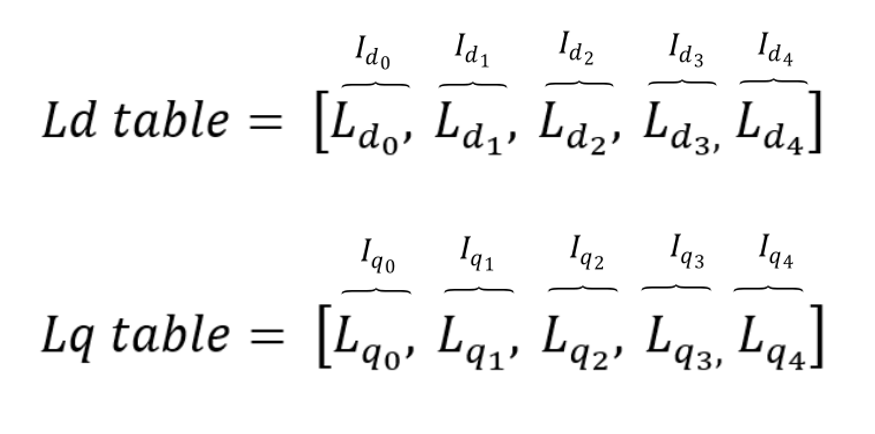Figure 3. Nonlinear Model Type: inductances as functions of stator currents, Ld=fd(id) , Lq=fq(iq)
id_vector = [-40.0, -20.0, 0.0, 20.0, 40.0]
iq_vector = [-40.0, -20.0, 0.0, 20.0, 40.0]
Ld_table = [0.00186383, 0.00325188, 0.00399657, 0.00136793, 0.000562082]
Lq_table = [0.00321572, 0.00538029, 0.00779154, 0.00535, 0.00319568]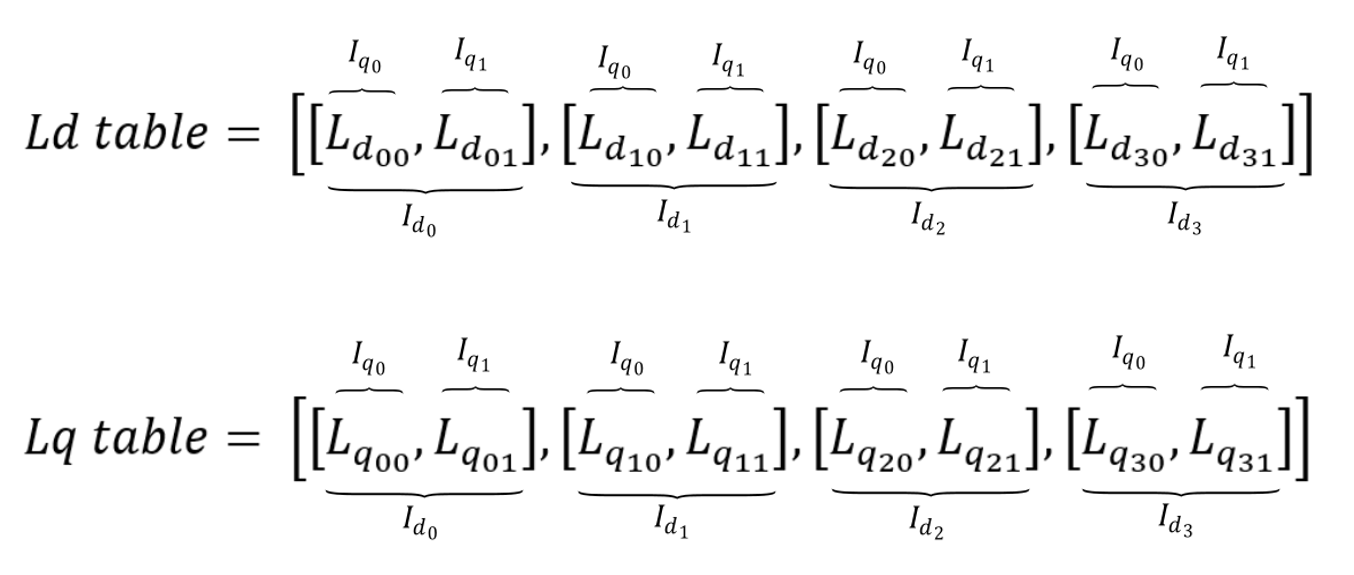Figure 4. Nonlinear Model Type: inductances as functions of stator currents, Ld=fd(id , iq) , Lq=fq(id , iq)
id_vector = [-40.0, -20.0, 0.0, 20.0, 40.0]
iq_vector = [-40.0, -20.0, 0.0, 20.0, 40.0]
Ld_table = [[0.00203118, 0.00188417, 0.00186383, 0.00188366, 0.00201026],
[0.00217976, 0.00297238, 0.00325188, 0.0029855, 0.00223459],
[0.00226518, 0.00283656, 0.00399657, 0.00280727, 0.00218666],
[0.0016353, 0.00171137, 0.00136793, 0.00178913, 0.00164534],
[0.00121342, 0.00096362, 0.000562082, 0.000967825, 0.00123179]]
Lq_table = [[0.00332706, 0.00419461, 0.0049565, 0.00419414, 0.00332745],
[0.00328404, 0.00520506, 0.00635444, 0.00520574, 0.00320567],
[0.00321572, 0.00538029, 0.00779154, 0.00535, 0.00319568],
[0.00293984, 0.00421955, 0.00547829, 0.00419697, 0.00290709],
[0.00273112, 0.00294274, 0.00323358, 0.00292902, 0.00271144]] 

## Mechanical

Table 5. Mechanical parameters
symbol description
pms Machine number of pole pairs
Star/delta Stator winding connection (star or delta)
Jm Combined rotor and load moment of inertia [kgm2]
Friction coefficient Machine viscous friction coefficient [Nms]
Unconstrained mechanical angle Limiting mechanical angle between 0 and 2π

symbol description
Load source Load can be set from SCADA/external or from model (in model case, one signal processing input will appear)
Load ai offset Assigned offset value to the input signal representing external torque command
Load ai gain Assigned gain value to the input signal representing external torque command

External load enables you to use an analog input signal from a HIL analog channel with the load_ai_pin address as an external torque/speed load, and to assign offset (V) and gain (Nm/V) to the input signal, according to the formula:

${T}_{l}=load_ai_gain·\left(AI\left(load_ai_pin\right)+load_ai_offset\right)$

Note: An analog input pin may be overwritten if another component uses the same analog input pin. If another property (from the same or a different component) uses the same analog input pin, the input signal value will be applied to only one of those properties. For instance, if both the load and the resolver carrier signal use the same analog input pin, the signal value will only be applied only to one of these.

## Feedback

Table 7. Feedback parameters
symbol description
Encoder ppr Incremental encoder number of pulses per revolution
Resolver pole pairs Resolver number of pole pairs
Resolver carrier source Resolver carrier signal source selection (internal or external)
External resolver carrier source type External resolver carrier signal source type selection (single ended or differential); available only if the Resolver carrier source property is set to external
Resolver carrier frequency Resolver carrier signal frequency (internal carrier) [Hz]
Resolver ai pin 1 Resolver carrier input channel 1 address (external carrier)
Resolver ai pin 2 Resolver carrier input channel 2 address (external carrier); available only if the External resolver carrier source type property is set to differential
Resolver ai offset Resolver carrier input channel offset (external carrier)
Resolver ai gain Resolver carrier input channel gain (external carrier)
Absolute encoder protocol Standardized protocol providing the absolute machine encoder position

If an external resolver carrier source is selected, the source signal type can be set as either single ended or differential. The single ended external resolver carrier source type enables use of an analog input signal from the HIL analog channel with the res_ai_pin_1 address as the external carrier source. Additionally, offset (V) and gain (V/V) values can be assigned to the input signal, according to the formula:

$res_carr_src=res_ai_gain·\left(AI\left(res_ai_pin_1\right)+res_ai_offset\right)$

The differential external resolver carrier source type enables use of two analog input signals from the HIL analog channels with the res_ai_pin_1 and the res_ai_pin_2 addresses. Analog signals from these HIL analog inputs are subtracted, and the resulting signal is used as the external differential carrier source. Additionally, offset (V) and gain (V/V) values can be assigned to the input signal (similarly to the single ended case), according to the formula:

$res_carr_src=res_ai_gain·\left(\left(AI\left(res_ai_pin_1\right)-AI\left(res_ai_pin_2\right)\right)+res_ai_offset\right)$
Note: Differential external resolver signal support is available from the 2023.1 software version. Only the single ended external resolver option was available in previous software versions.
Note: In order to get a resolver signal with an amplitude of 1 when using an external carrier signal, the offset and the gain should be chosen in such a way that the resolver carrier signal has an amplitude of 1 after the adjustment. As shown in Figure 1, the sinusoidal signal used to generate external resolver carrier source is fed to HIL analog input 1. The analog input signal is scaled in order to get the resolver signals with an amplitude of 1.
Note: An analog input pin may be overwritten if another component uses the same analog input pin. If another property (from the same or a different component) uses the same analog input pin, the input signal value will be applied to only one of those properties. E.g. if both the load and the resolver carrier signal use the same analog input pin, the signal value will be applied only to one of these.

The following expression must hold in order to properly generate the encoder signals:

$4·enc_ppr·{f}_{m}{·T}_{s}\le 1$
Table 8. Variables in the encoder limitation expression
symbol description
enc_ppr Encoder number of pulses per revolution
fm Rotor mechanical frequency [Hz]
Ts Simulation time step [s]
Note: While the machine speed is positive, the encoder channel B signal leads the encoder channel A signal.
Note: Absolute encoder protocol is not supported on HIL402 (configurations: 1, 2, 3, and 4).

symbol description
Theta_ab Position of the stationary αβ reference frame, in respect to the stator phase a axis [rad]

The machine model output variables (currents, voltages and fluxes) can be observed from a stationary reference frame. There are two widely used approaches in electrical machine modeling: in the first, the alpha axis of the stationary reference frame lags by 90 degrees in regard to the stator phase a axis (used by default, and indicated in a) Figure 5. In the second one, the alpha axis is aligned with the stator phase a axis (indicated in b) Figure 5. The user can select between these two situations.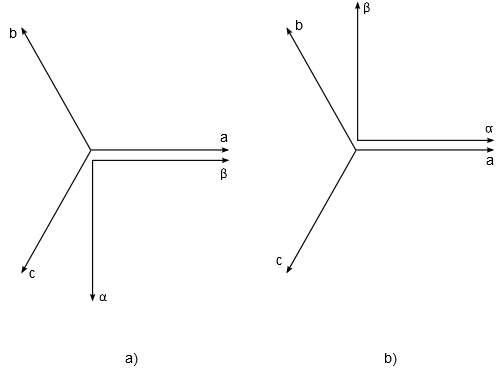Figure 7. Selection of a stationary reference frame position: a) Theta_ab= -pi/2; b) Theta_ab= 0

It is important to know the value of Theta_ab when the rotor position feedback is necessary. As an example, if a model uses the mechanical angle as a feedback signal and feeds it to one of the abc to dq, alpha beta to dq, dq to abc, or dq to alpha beta transformation blocks, the same transformation angle offset value should be used in both components to ensure the expected simulation results.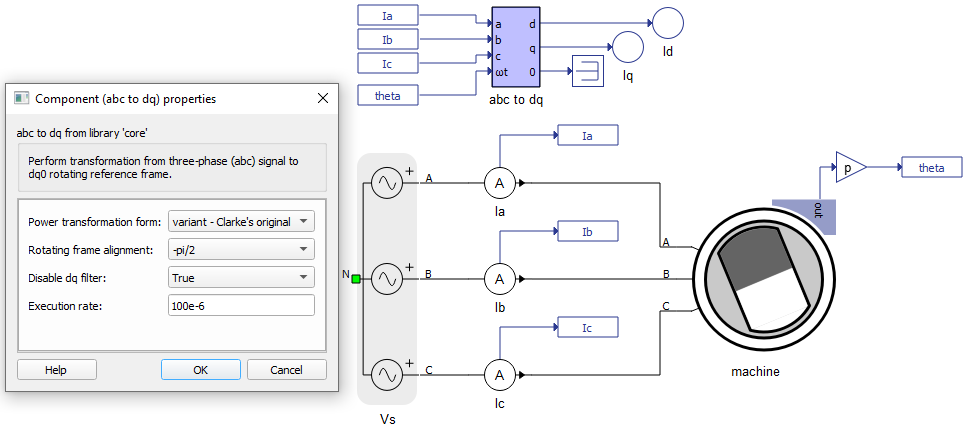Figure 8. Example of model containing abc to dq transformation component and chosen stationary reference frame position Theta_ab= -pi/2
Note: This property is available only on certain machine components.

## Output

This block tab enables a single, vectorized signal output from the machine. The output vector contains selected machine mechanical and/or electrical variables in the same order as listed in this tab.

Note: All machine components have the Execution rate, Electrical torque, Mechanical speed, and Mechanical angle, but the rest of the signals differ from component to component.
Table 10. Output parameters
symbol description
Execution rate Signal processing output execution rate [s]
Electrical torque Machine electrical torque [Nm]
Mechanical speed Machine mechanical angular speed [rad/s]
Mechanical angle Machine mechanical angle [rad]
Stator alpha axis current Alpha axis component of the stator current [A]
Stator beta axis current Beta axis component of the stator current [A]
Stator d-axis current Direct axis component of the stator current [A]
Stator q-axis current Quadrature axis component of the stator current [A]
Stator alpha axis flux Alpha axis component of the stator flux [Wb]
Stator beta axis flux Beta axis component of the stator flux [Wb]
Stator d-axis flux Direct axis component of the stator flux [Wb]
Stator q-axis flux Quadrature axis component of the stator flux [Wb]